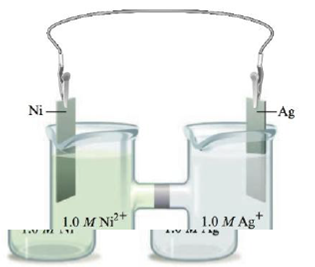# Consider the following galvanic cell: Calculate the concentrations of Ag + ( aq ) and Ni 2+ ( aq ) once the cell is “dead.”### Chemistry: An Atoms First Approach

2nd Edition
Steven S. Zumdahl + 1 other
Publisher: Cengage Learning
ISBN: 9781305079243

#### Solutions

Chapter
Section### Chemistry: An Atoms First Approach

2nd Edition
Steven S. Zumdahl + 1 other
Publisher: Cengage Learning
ISBN: 9781305079243
Chapter 17, Problem 151CP
Textbook Problem
1 views

## Consider the following galvanic cell:Calculate the concentrations of Ag+(aq) and Ni2+(aq) once the cell is “dead.”

Interpretation Introduction

Interpretation:

The set up of a galvanic cell having Silver and Nickel electrode is given. The concentration of Ag+(aq) and Ni2+(aq) once the given cell is dead is to be calculated.

Concept introduction:

The equilibrium constant is used to determine the direction of the equilibrium. The higher value of K corresponds to the forward reaction and the lower value of K corresponds to the backward reaction.

To determine: The concentration of Ag+(aq) and Ni2+(aq) once the given cell is dead.

The value of K for the given cell reaction is 7.24×1034_ .

### Explanation of Solution

The reaction taking place at the cathode is,

Ag++eAgE°red=0.80V

The reaction taking place at the anode is,

NiNi2++2eE°ox=0.23V

Multiply the reduction half-reaction with a coefficient of 2 and then add both the reduction and oxidation half-reactions as,

2Ag++2e2AgNiNi2++2e

The final equation is,

Ni+2Ag+2Ag+Ni2+

The value of E°cell is given as,

cell=E°ox+E°red

Where,

• ox is the oxidation potential of the electrode.
• red is the reduction potential of the electrode.

Substitute the values of E°ox and E°red in the above equation as,

cell=E°ox+E°red=0.23V+0.80V=1.03V

The cell potential is calculated using the Nernst equation,

E=E°-(0.0591n)log(Q)

Where,

• E is the cell potential.
• is the cell potential at the standard conditions.
• Q is the activity of the species in the cell.
• n is the number of electrons involved in the reaction.

As the cell is dead, it means the reaction has reached equilibrium. Therefore, at equilibrium, the value of Q is replaced with the equilibrium constant K and at equilibrium the value of E is equal to zero.

The reaction involves the transfer of 2 moles of electrons.

Rearrange the equation to obtain the value of E° as,

E°=E-(0.0591n)log(K)

Substitute the values of E , E° , n , in the above equation as,

E=E°-(0.0591n)log(K)0-1.03=-(0.05912)log(K)log(K)=34.86K=1034.86

The equation is further simplified as,

K=1034.86=7.24×1034_

The value of K for the given cell reaction is 7.24×1034_ .

The concentration of Ag+ from the reverse reaction is 0.46×10-17M_ and the concentration of Ni2+ from the reverse reaction is 1.5M_ .

The value of K for the given cell reaction is 7.24×1034_ .

As the value of equilibrium constant is high, it means that the reaction is about to complete.

Make the ICE table for the given cell reaction,

2Ag++Ni2Ag+Ni2+Initial:1

### Still sussing out bartleby?

Check out a sample textbook solution.

See a sample solution

#### The Solution to Your Study Problems

Bartleby provides explanations to thousands of textbook problems written by our experts, many with advanced degrees!

Get Started

Find more solutions based on key concepts
The appetite-stimulating hormone ghrelin is made by the a. brain b. fat tissue c. pancreas d. stomach

Nutrition: Concepts and Controversies - Standalone book (MindTap Course List)

Earth did not rotate, could you define the celestial poles and celestial equator?

Horizons: Exploring the Universe (MindTap Course List)

What is the structure and function of a chloroplast?

Biology: The Dynamic Science (MindTap Course List)

This problem describes one experimental method for determining the moment of inertia of an irregularly shaped o...

Physics for Scientists and Engineers, Technology Update (No access codes included)

What happens at each?

Oceanography: An Invitation To Marine Science, Loose-leaf Versin